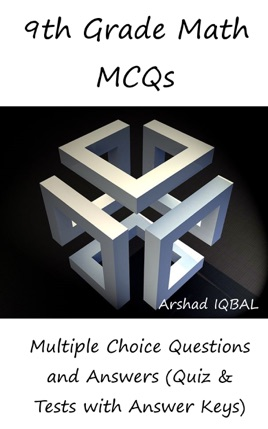• 3,99 €

## Descrizione dell’editore

"9th Grade Math MCQs: Multiple Choice Questions and Answers (Quiz & Tests with Answer Keys)" provides mock tests for competitive exams preparation. "Grade 9 Math MCQ" pdf to download helps with theoretical, conceptual, and analytical study for self-assessment, career tests. 9th grade math quizzes, a quick study guide can help to learn and practice questions for placement test preparation.
"Grade 9 Math Multiple Choice Questions and Answers (MCQs)" pdf to download is a revision guide with a collection of trivia questions to fun quiz questions and answers pdf on topics: Algebraic expressions and algebraic formulas, algebraic manipulation, arithmetic and geometric sequences, basic math problems, basic statistics, business mathematics, congruent triangles and geometry, consumer math, factorization, introduction to logarithms, linear equations and inequalities, linear graphs and applications, logarithms and exponents, mathematical theorems, matrices and determinants, percentage, ratio and proportion, real and complex numbers, sets and functions to enhance teaching and learning.
Grade 9 Math Quiz Questions and Answers pdf also covers the syllabus of many competitive papers for admission exams of different schools from math textbooks on chapters:

Algebraic Expressions and Algebraic Formulas Multiple Choice Questions: 49 MCQs. Algebraic Manipulation Multiple Choice Questions: 13 MCQs. Arithmetic and Geometric Sequences Multiple Choice Questions: 36 MCQs. Basic Math Problems Multiple Choice Questions: 20 MCQs. Basic Statistics Multiple Choice Questions: 35 MCQs. Business Mathematics Multiple Choice Questions: 37 MCQs. Congruent Triangles and Geometry Multiple Choice Questions: 17 MCQs. Consumer Math Multiple Choice Questions: 20 MCQs. Factorization Multiple Choice Questions: 13 MCQs. Introduction to Logarithms Multiple Choice Questions: 30 MCQs. Linear Equations and Inequalities Multiple Choice Questions: 27 MCQs. Linear Graphs and Applications Multiple Choice Questions: 16 MCQs. Logarithms and Exponent.s Multiple Choice Questions: 12 MCQs. Mathematical Theorems Multiple Choice Questions: 24 MCQs. Matrices and Determinants Multiple Choice Questions: 48 MCQs. Percentage, Ratio and Proportion Multiple Choice Questions: 51 MCQs. Real and Complex Numbers Multiple Choice Questions: 61 MCQs. Sets and Functions Multiple Choice Questions: 30 MCQs.

"Algebraic Expressions and Algebraic Formulas MCQ" pdf chapter covers quiz about algebraic expressions, algebra formulas, and rationalization of surds.
"Algebraic Manipulation MCQ" pdf chapter covers quiz about square root of algebraic expression, LCM, and HCF.
"Basic Statistics MCQ" pdf chapter covers quiz about central tendency measurements, central tendency: mean, median and mode, measures of central tendency, cumulative frequency, frequency distribution, and measures of dispersion.